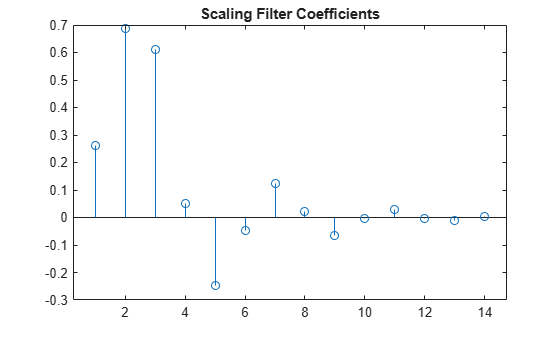fejerkorovkin

Fejér-Korovkin wavelet filters

Description

example

Lo = fejerkorovkin(wname) returns the Fejér-Korovkin scaling filter specified by wname. Valid entries for wname are 'fk4', 'fk6', 'fk8', 'fk14', 'fk18', and 'fk22'. For information on the Fejér–Korovkin filters, see Nielson.

Examples

collapse all

Construct and plot the Fejer-Korovkin (14) scaling function and wavelet.

Obtain the Fejer-Korovkin scaling filter and display its 14 coefficients.

Lo = fejerkorovkin('fk14')
Lo = 1×14

0.2604    0.6869    0.6116    0.0514   -0.2456   -0.0486    0.1243    0.0222   -0.0640   -0.0051    0.0298   -0.0033   -0.0093    0.0035

Use the scaling filter to obtain the wavelet filter and display its wavelet filter coefficients.

Hi = qmf(Lo)
Hi = 1×14

0.0035    0.0093   -0.0033   -0.0298   -0.0051    0.0640    0.0222   -0.1243   -0.0486    0.2456    0.0514   -0.6116    0.6869   -0.2604

wavefun provides an efficient way to construct and plot the scaling function and wavelet.

[phi,psi,xval] = wavefun('fk14');
subplot(2,1,1)
plot(xval,phi)
title('Scaling Function')
subplot(2,1,2)
plot(xval,psi)
title('Wavelet')Input Arguments

collapse all

Name of desired Fejér-Korovkin scaling filter. The numeric value in each name is the number of Fejér-Korovkin filter coefficients. For example, if wname is 'fk14', Lo has 14 coefficients.

Output Arguments

collapse all

Scaling filter, returned as a vector.

 Nielsen, M. "On the construction and frequency localization of finite orthogonal quadrature filters." Journal of Approximation Theory. Vol. 108, Number 1, 2001, pp. 36–52.

Wavelet Toolbox DocumentationGet trial now